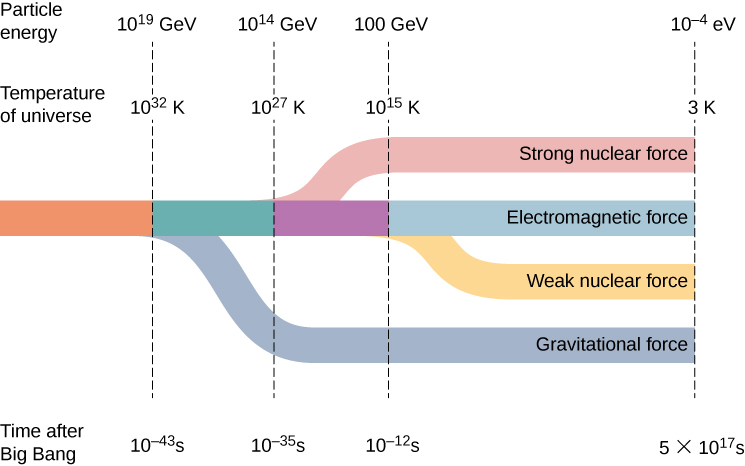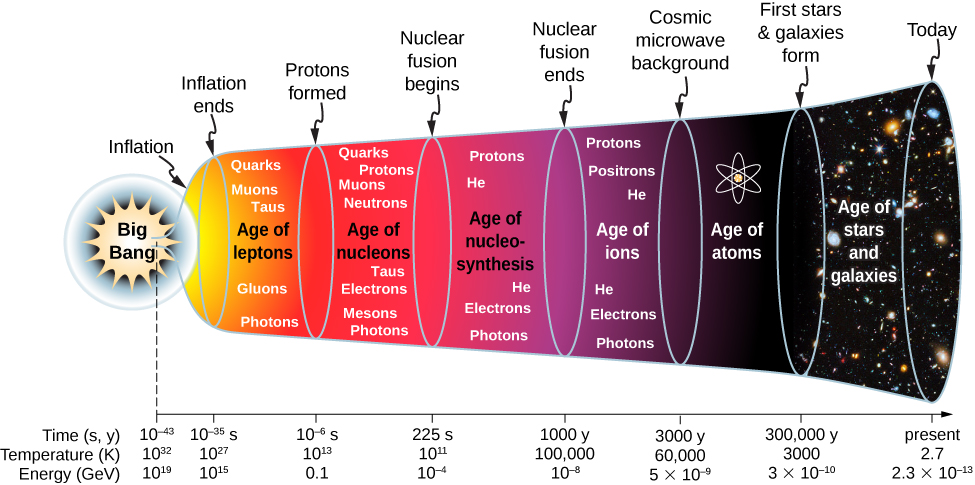# 11.7 Evolution of the early universe

 Page 1 / 10
By the end of this section, you will be able to:
• Describe the evolution of the early universe in terms of the four fundamental forces
• Use the concept of gravitational lensing to explain astronomical phenomena
• Provide evidence of the Big Bang in terms of cosmic background radiation
• Distinguish between dark matter and dark energy

In the previous section, we discussed the structure and dynamics of universe. In particular, the universe appears to be expanding and even accelerating. But what was the universe like at the beginning of time? In this section, we discuss what evidence scientists have been able to gather about the early universe and its evolution to present time.

## The early universe

Before the short period of cosmic inflation, cosmologists believe that all matter in the universe was squeezed into a space much smaller than an atom. Cosmologists further believe that the universe was extremely dense and hot, and interactions between particles were governed by a single force. In other words, the four fundamental forces (strong nuclear, electromagnetic, weak nuclear, and gravitational) merge into one at these energies ( [link] ). How and why this “unity” breaks down at lower energies is an important unsolved problem in physics.The separation of the four fundamental forces in the early universe.

Scientific models of the early universe are highly speculative. [link] shows a sketch of one possible timeline of events.An approximate timeline for the evolution of the universe from the Big Bang to the present.
1. Big Bang $\left(t<1{0}^{\text{−}43}\text{s}\right):$ The current laws of physics break down. At the end of the initial Big Bang event, the temperature of the universe is approximately $T=1{0}^{32}\text{K}.$
2. Inflationary phase The universe expands exponentially, and gravity separates from the other forces. The universe cools to approximately $T=1{0}^{27}\text{K}.$
3. Age of leptons As the universe continues to expand, the strong nuclear force separates from the electromagnetic and weak nuclear forces (or electroweak force). Soon after, the weak nuclear force separates from the electromagnetic force. The universe is a hot soup of quarks, leptons, photons, and other particles.
4. Age of nucleons The universe consists of leptons and hadrons (such as protons, neutrons, and mesons) in thermal equilibrium. Pair production and pair annihilation occurs with equal ease, so photons remain in thermal equilibrium:
$\begin{array}{}\\ \\ \gamma +\gamma ↔{\text{e}}^{\text{−}}+{\text{e}}^{+}\hfill \\ \gamma +\gamma ↔\text{p}+\stackrel{\text{−}}{\text{p}}\hfill \\ \gamma +\gamma ↔\phantom{\rule{0.2em}{0ex}}\text{n}+\stackrel{\text{−}}{\text{n}}.\hfill \end{array}$

The number of protons is approximately equal to the number of neutrons through interactions with neutrinos:
$\begin{array}{}\\ \\ {\nu }_{e}+\text{n}\phantom{\rule{0.2em}{0ex}}↔{\text{e}}^{\text{−}}+\text{p}\hfill \\ {\stackrel{\text{−}}{\nu }}_{e}+\text{p}\phantom{\rule{0.2em}{0ex}}↔{\text{e}}^{+}+\text{n.}\hfill \end{array}$

The temperature of the universe settles to approximately $1{0}^{11}\text{K}$ —much too cool for the continued production of nucleon-antinucleon pairs. The numbers of protons and neutrons begin to dominate over their anti-particles, so proton-antiproton $\left(\text{p}\stackrel{\text{−}}{\text{p}}\right)$ and neutron-antineutron ( $\text{n}\stackrel{\text{−}}{\text{n}}$ ) annihilations decline. Deuterons (proton-neutron pairs) begin to form.
5. Age of nucleosynthesis ( $t={225}^{}\text{s}$ to 1000 years): As the universe continues to expand, deuterons react with protons and neutrons to form larger nuclei; these larger nuclei react with protons and neutrons to form still larger nuclei. At the end of this period, about 1/4 of the mass of the universe is helium. (This explains the current amount of helium in the universe.) Photons lack the energy to continue electron-positron production, so electrons and positrons annihilate each other to photons only.
6. Age of ions ( $t=1000$ to 3000 years): The universe is hot enough to ionize any atoms formed. The universe consists of electrons, positrons, protons, light nuclei, and photons.
7. Age of atoms ( $t=3000$ to 300,000 years): The universe cools below ${10}^{5}\text{K}$ and atoms form. Photons do not interact strongly with neutral atoms, so they “decouple” (separate) from atoms. These photons constitute the cosmic microwave background radiation to be discussed later.
8. Age of stars and galaxies ( $t=300,000$ years to present): The atoms and particles are pulled together by gravity and form large lumps. The atoms and particles in stars undergo nuclear fusion reaction.

#### Questions & Answers

in the wave equation y=Asin(kx-wt+¢) what does k and w stand for.
derivation of lateral shieft
Hi
Hi
hi
ALFRED
how are you?
hi
asif
hi
Imran
I'm fine
ALFRED
total binding energy of ionic crystal at equilibrium is
How does, ray of light coming form focus, behaves in concave mirror after refraction?
Refraction does not occur in concave mirror. If refraction occurs then I don't know about this.
Sushant
What is motion
Anything which changes itself with respect to time or surrounding
Sushant
good
Chemist
and what's time? is time everywhere same
Chemist
No
Sushant
how can u say that
Chemist
do u know about black hole
Chemist
Not so more
Sushant
DHEERAJ
These substance create harmful radiation like alpha particle radiation, beta particle radiation, gamma particle radiation
Sushant
But ask anything changes itself with respect to time or surrounding A Not any harmful radiation
DHEERAJ
explain cavendish experiment to determine the value of gravitational concept.
For the question about the scuba instructor's head above the pool, how did you arrive at this answer? What is the process?
as a free falling object increases speed what is happening to the acceleration
of course g is constant
Alwielland
acceleration also inc
Usman
which paper will be subjective and which one objective
jay
normal distributiin of errors report
Dennis
normal distribution of errors
Dennis
photo electrons doesn't emmit when electrons are free to move on surface of metal why?
What would be the minimum work function of a metal have to be for visible light(400-700)nm to ejected photoelectrons?
give any fix value to wave length
Rafi
40 cm into change mm
40cm=40.0×10^-2m =400.0×10^-3m =400mm. that cap(^) I have used above is to the power.
Prema
i.e. 10to the power -2 in the first line and 10 to the power -3 in the the second line.
Prema
there is mistake in my first msg correction is 40cm=40.0×10^-2m =400.0×10^-3m =400mm. sorry for the mistake friends.
Prema
40cm=40.0×10^-2m =400.0×10^-3m =400mm.
Prema
this msg is out of mistake. sorry friends​.
Prema
what is physics?
why we have physics
because is the study of mater and natural world
John
because physics is nature. it explains the laws of nature. some laws already discovered. some laws yet to be discovered.
Yoblaze
physics is the study of non living things if we added it with biology it becomes biophysics and bio is the study of living things tell me please what is this?
tahreem
physics is the study of matter,energy and their interactions
Buvanes
all living things are matter
Buvanes
why rolling friction is less than sliding friction
tahreem
thanks buvanas
tahreem
is this a physics forum
explain l-s couplingByBy Dravida Mahadeo-J...By